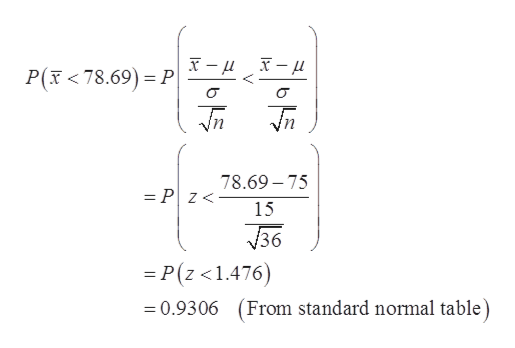# MNM Corporation gives each of its 500 employees an aptitude test. The scores on the test are normally distributed with a mean of 75 and a standard deviation of 15. A simple random sample of 36 is taken from the population of 500 employees. Using only the appropriate statistical table in your textbook, what is the probability that the average aptitude test score in the sample will be less than 78.69?ANSWER:  (Report your final answer to 4 decimal places, using conventional rounding rules) Using only the appropriate statistical table in your textbook, four percent of the samples of n = 36 taken from this population should have a mean test score above what value?ANSWER:  (Report your final answer to 4 decimal places, using conventional rounding rules)

Question
68 views

MNM Corporation gives each of its 500 employees an aptitude test. The scores on the test are normally distributed with a mean of 75 and a standard deviation of 15. A simple random sample of 36 is taken from the population of 500 employees.

Using only the appropriate statistical table in your textbook, what is the probability that the average aptitude test score in the sample will be less than 78.69?

Using only the appropriate statistical table in your textbook, four percent of the samples of n = 36 taken from this population should have a mean test score above what value?

check_circle

Step 1

The provided information are:

Step 2

Consider, X be the random variable that represents the test scores is normally distributed with a mean of 75 and a standard deviation of...help_outlineImage TranscriptioncloseP(x <78.69) P 78.69-75 = Pz- 15 36 P(z <1.476) From standard normal table) =0.9306 fullscreen

### Want to see the full answer?

See Solution

#### Want to see this answer and more?

Solutions are written by subject experts who are available 24/7. Questions are typically answered within 1 hour.*

See Solution
*Response times may vary by subject and question.
Tagged in

### Other